# 5 Draw a computational graph to compute the function f(, y) ( graph to compute f (2,3) -y). Use the Draw a reverse mode graph to compute the derivatives f/0x and อ//ay for f 3( y). Use the graph...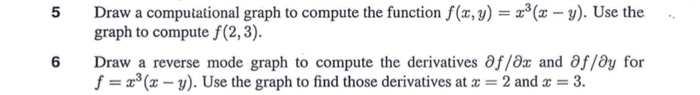5 Draw a computational graph to compute the function f(, y) ( graph to compute f (2,3) -y). Use the Draw a reverse mode graph to compute the derivatives f/0x and อ//ay for f 3( y). Use the graph to find those derivatives at 2 and 3. 6Consider the equationTo draw the computational graph, we simplify the equation into it's constituent terms i.e.,

Let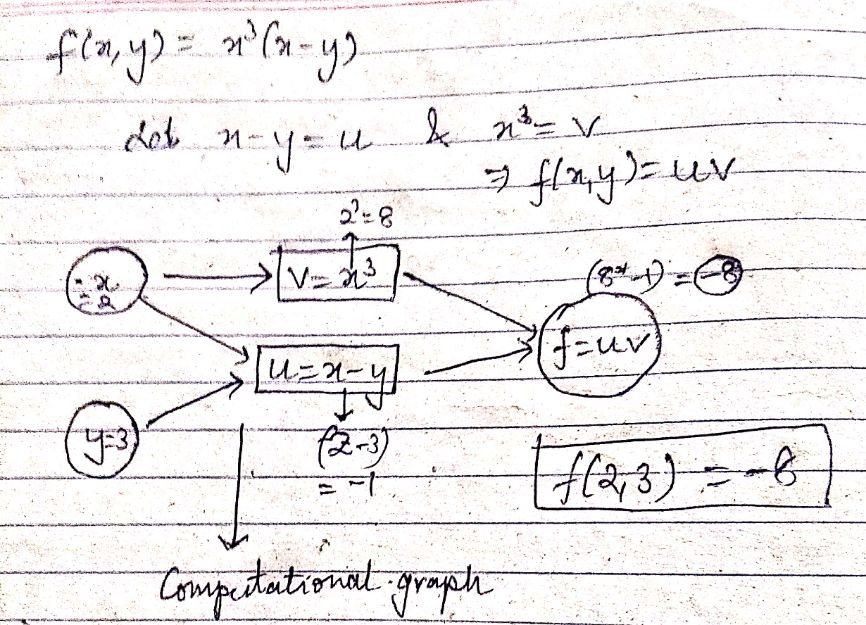So,For the reverse-mode graph, first we compute the partial derivatives :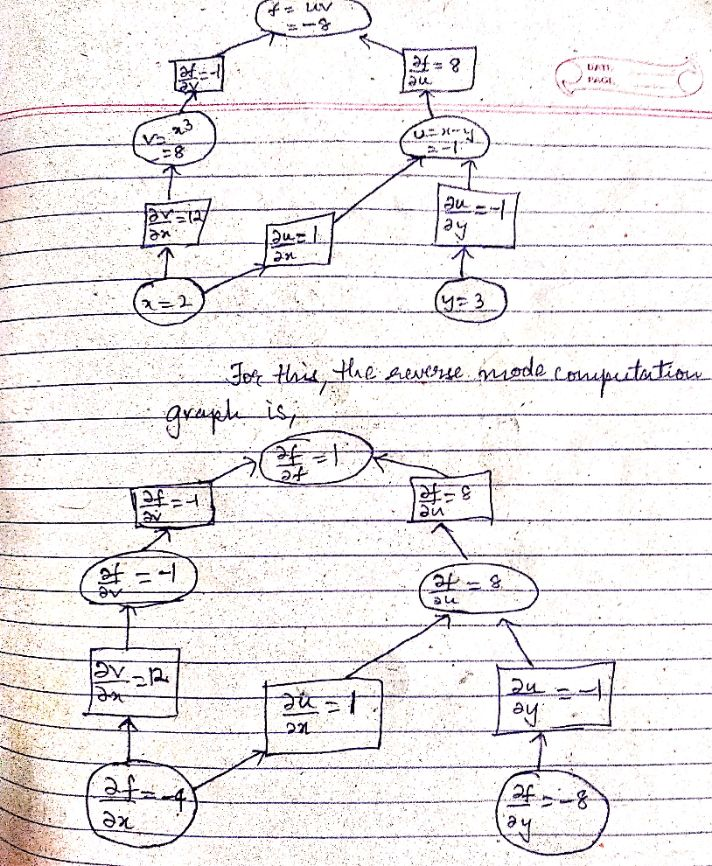##### Add Answer of: 5 Draw a computational graph to compute the function f(, y) ( graph to compute f (2,3) -y). Use the Draw a reverse mode graph to compute the derivatives f/0x and อ//ay for f 3( y). Use the graph...
Similar Homework Help Questions
• ### 5. Given to the right is the graph of the function f(r, y) ye/2 on the...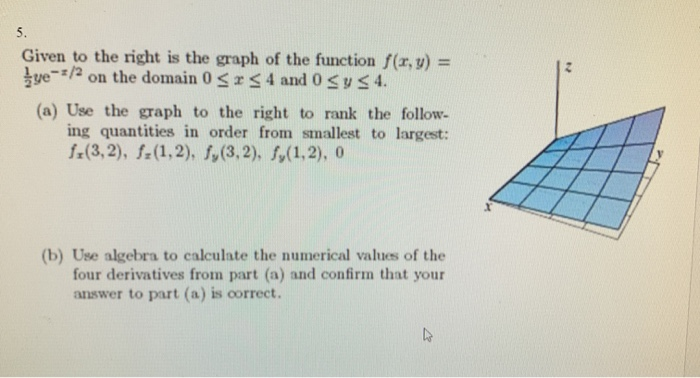5. Given to the right is the graph of the function f(r, y) ye/2 on the domain 0r4 and 0 ys 4 (a) Use the graph to the right to rank the follow ing quantities in order from smallest to largest: f(3,2), f(1,2), f,(3,2), f,(1,2), 0 (b) Use algebra to calculate the numerical values of the four derivatives from part (a) and confirm that your answer to part (a) is correct. 5. Given to the right is the graph of...

• ### QUESTION 8 Find all the first order partial derivatives for the following function. fx, y) x-9x2y- 10xy 3 a x29xy-1092102 ах b. af ー= 3x2, ах af 9x2-3 OC.df =3x2 + 2xy . 10y3 =-9x2 + 3xy2 ay d....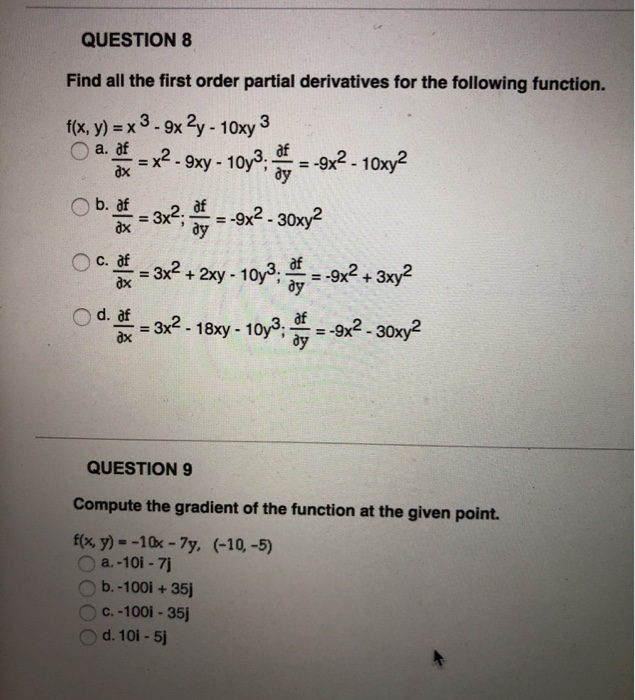QUESTION 8 Find all the first order partial derivatives for the following function. fx, y) x-9x2y- 10xy 3 a x29xy-1092102 ах b. af ー= 3x2, ах af 9x2-3 OC.df =3x2 + 2xy . 10y3 =-9x2 + 3xy2 ay d. af QUESTION 9 Compute the gradient of the function at the given point. ffx y)--10x-7y, (-10-5) O b.-100i+35 c.-100i-35j d. 10i - 5 QUESTION 8 Find all the first order partial derivatives for the following function. fx, y) x-9x2y- 10xy 3...

• ### 2. Consider the following function: Compute each of the following: Hint: There is probably a better way to compute these than to just mindlessly compute all ot partial derivatives in the order gi...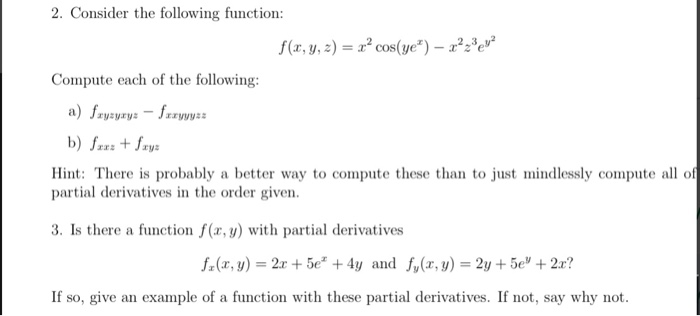2. Consider the following function: Compute each of the following: Hint: There is probably a better way to compute these than to just mindlessly compute all ot partial derivatives in the order given 3. Is there a function f(x, y) with partial derivatives f, (z, y) = 2r + 5e" + 4y and f,(x, y) = 2y + 5e" + 2x? If so, give an example of a function with these partial derivatives. If not, say why not 2. Consider...

• ### [Question 1] Find and graph the domain of the function f(,y)-In-) Question 2] Graph a contour map...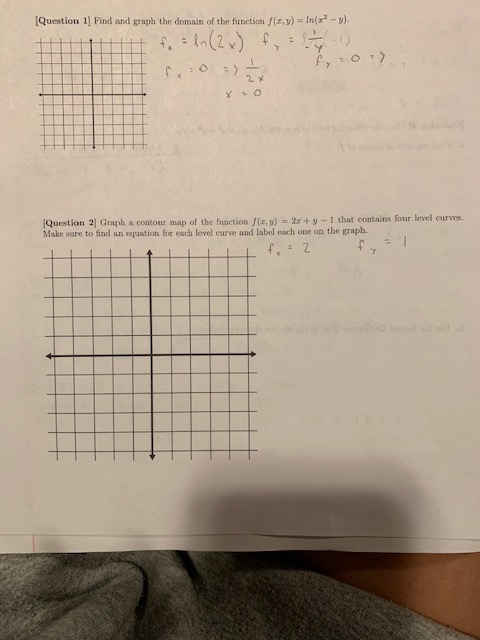[Question 1] Find and graph the domain of the function f(,y)-In-) Question 2] Graph a contour map of the function f(z, y)2s y 1 that contains four level curves. Make sure to find an equation for each level curve and label each one on the graph. IQuestion 3] The equation of the tangeat plane to the function z the equation: Using the form of the equatioa above, fiud the tangent plane to f(a,y)yat the point (2. ). Question 4] Find...

• ### 3. For each function, use the differential to evaluate Ay due to the given changes inr Assume tha...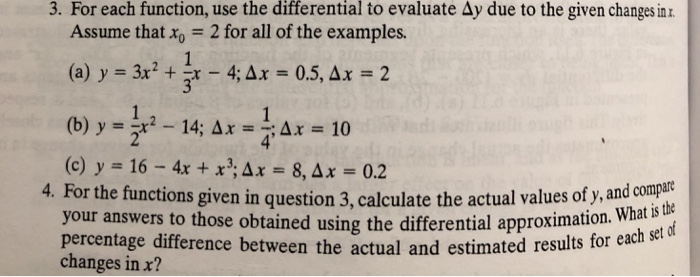do #4 with # 3 question 3. For each function, use the differential to evaluate Ay due to the given changes inr Assume that x 2 for all of the examples. (a) y = 3x2 + 1x-4;Ax = 0.5, Ax-2 (b) y- 14; AxAr 10 (c) y = 16-4x + x,Ax=8,Ax=0.2 your answers to those obtained using the differential approximatin wih set df 4. For the functions given in question 3, calculate the actual values of y, and compat percentage...

• ### 3. Shape of a Graph Below are the graphs of the first and second derivatives of a function f. Estimate the coordinate of the point of inflection and reconstruct the graph of f. You do not need to dra...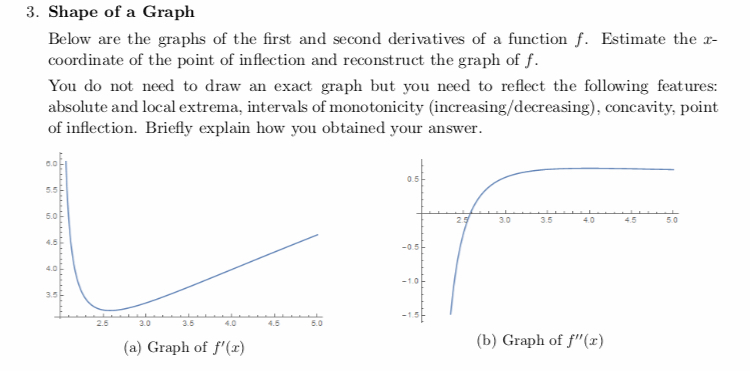3. Shape of a Graph Below are the graphs of the first and second derivatives of a function f. Estimate the coordinate of the point of inflection and reconstruct the graph of f. You do not need to draw an exact graph but you need to reflect the following features: absolute and local extrema, intervals of monotonicity (increasing/decreasing), concavity, point of inflection. Briefly explain how you obtained your answer. 0.0 0.5 5.0 30 35 40 4550 4.5 -0.5 1.0 3.5...

• ### 6. For the function y = X1 X2 find the partial derivatives by using definition 11.1. (w) with respect to the Defin...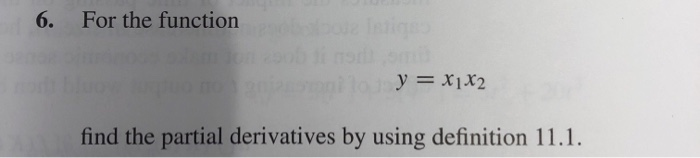6. For the function y = X1 X2 find the partial derivatives by using definition 11.1. (w) with respect to the Definition 11.1 The partial derivative of a function y = f(x1,x2,...,xn) with respe variable x; is af f(x1, ..., X; + Axi,...,xn) – f(x1,...,,.....) axi Ax0 ΔΧ The notations ay/ax, or f(x) or simply fare used interchangeably. Notice that in defining the partial derivative f(x) all other variables, x;, j i, are held constant As in the case of...

• ### 1 Let f (z, y)5) Find the equation for the tangent plane to the graph of f at the point (3, 3) (Use symbolic notation a...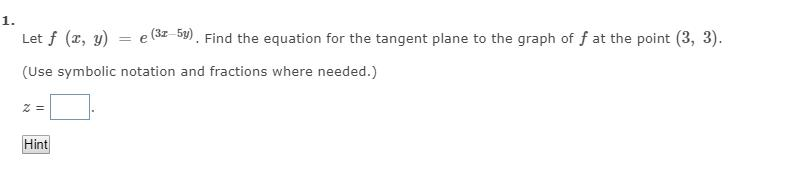1 Let f (z, y)5) Find the equation for the tangent plane to the graph of f at the point (3, 3) (Use symbolic notation and fractions where needed.) Hint 1 Let f (z, y)5) Find the equation for the tangent plane to the graph of f at the point (3, 3) (Use symbolic notation and fractions where needed.) Hint

• ### 72 Partial Derivatives: Problem 16 Next Previous Problem List (1 point) Suppose the f(x, y) is a smooth function and th...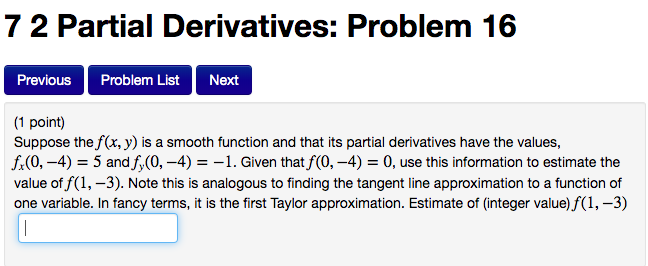72 Partial Derivatives: Problem 16 Next Previous Problem List (1 point) Suppose the f(x, y) is a smooth function and that its partial derivatives have the values, f(0,-4) 5 and f,(0, -4) =-1. Given that f(0,-4) = 0, use this information to estimate the value of f(1,-3) Note this is analogous to finding the tangent line approximation to a function of one variable. In fancy terms, it is the first Taylor approximation. Estimate of (integer value) f(1,-3) 72 Partial Derivatives:...

• ### Question 2 (20 points): Consider the functions f(x, y)-xe y sin y and g(x, y)-ys 1. Show f is differentiable in its domain 2. Compute the partial derivatives of g at (0,0) 3. Show that g is not d...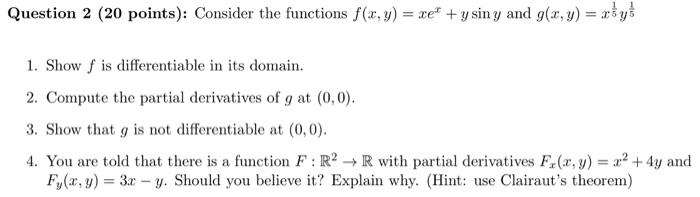Question 2 (20 points): Consider the functions f(x, y)-xe y sin y and g(x, y)-ys 1. Show f is differentiable in its domain 2. Compute the partial derivatives of g at (0,0) 3. Show that g is not differentiable at (0,0) 4. You are told that there is a function F : R2 → R with partial derivatives F(x,y) = x2 +4y and Fy(x, y 3x - y. Should you believe it? Explain why. (Hint: use Clairaut's theorem) Question 2...

Need Online Homework Help?